Back

Bézier Curves
Urs Oswald    osurs@bluewin.ch    http://www.ursoswald.ch
September 11, 2002

 Quadratic Bézier curves Cubic Bézier curves Bézier curves of arbitrary order

 Let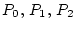be distinct points. If points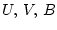divide the line segments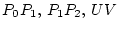by an equal ratio, then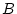moves on a quadratic Bézier curve if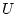moves about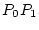. Thus for some real number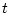,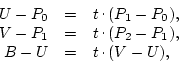where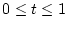.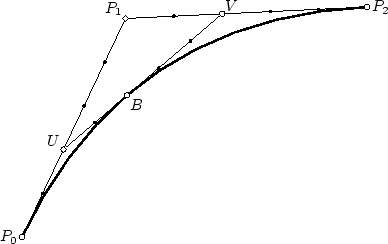Fig. 1: Quadratic Bézier curve

From the above equations, it follows that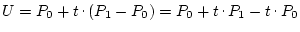, therefore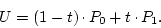(1)

We equally find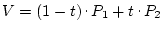and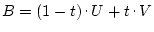. Substitution yields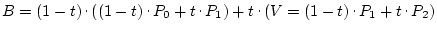, and after expansion,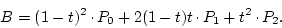(2)

## Cubic Bézier curves

 Let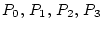be distinct points, and let the points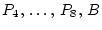divide their respective line segments by an equal ratio: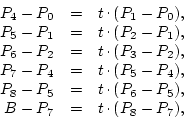where. (In fig. 2 as well as in fig. 1,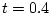.)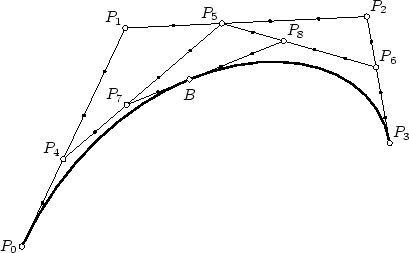Fig. 2: Cubic Bézier curve

From (2), we get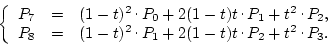From (1),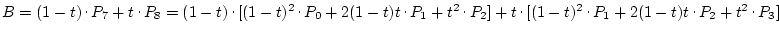which, after expansion, yields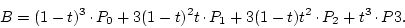(3)

In fig. 2, if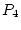moves about the segment, then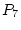moves on the quadratic Bézier curve determined by points, while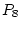moves on the quadratic Bézier curve determined by points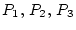.

## Bézier curves of arbitrary order

For distinct points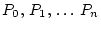, the Bézier curve of order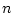(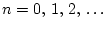) can be recursively defined by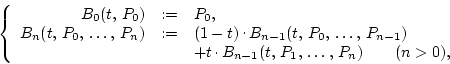(4)

where.

Theorem 1 (Bézier curves of order 1)   For points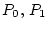, the Bézier curve of order 1 is given by the equation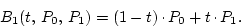PROOF: By the above definition,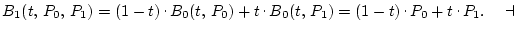Theorem 2   For any non-negative integer, the Bézier curve of orderis given by the equation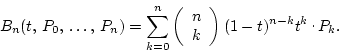PROOF: By induction on. For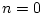, the theorem claims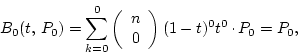which is correct by the first part of the definition.
For, we have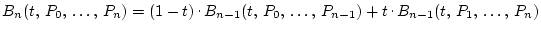by definition. By induction hypothesis,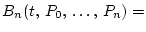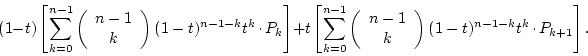We get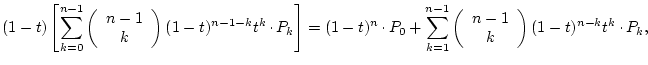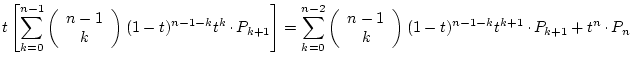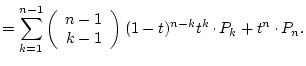Substituting the last two results, we get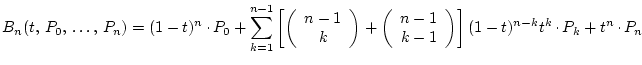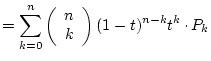,        as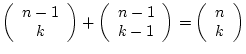by the fundamental law of the binomial coefficients.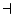Quadratic Bézier curves Cubic Bézier curves Bézier curves of arbitrary order